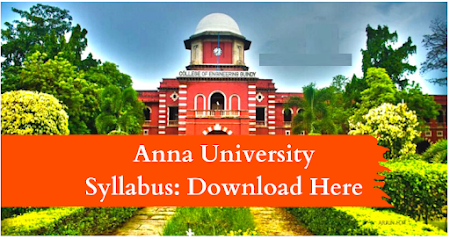### MA8351   DISCRETE MATHEMATICS     L    T    P  C   4    0    0    4

OBJECTIVES:  To extend student‘s logical and mathematical maturity and ability to deal with abstraction.  To introduce most of the basic terminologies used in computer science courses and application of ideas to solve practical problems.  To understand the basic concepts of combinatorics and graph theory.  To familiarize the applications of algebraic structures.  To understand the concepts and significance of lattices and boolean algebra which are widely used in computer science and engineering.

UNIT I   LOGIC AND PROOFS                                                                                                      12  Propositional logic – Propositional equivalences - Predicates and quantifiers – Nested quantifiers – Rules of inference - Introduction to proofs – Proof methods and strategy.

UNIT II COMBINATORICS                                                                                                            12  Mathematical induction – Strong induction and well ordering – The basics of counting – The pigeonhole principle – Permutations and combinations – Recurrence relations – Solving linear recurrence relations – Generating functions – Inclusion and exclusion principle and its applications

UNIT III GRAPHS                                                                                                                             12 Graphs and graph models – Graph terminology and special types of graphs – Matrix representation of graphs and graph isomorphism – Connectivity – Euler and Hamilton paths.

UNIT IV        ALGEBRAIC STRUCTURES                                                                                   12 Algebraic  systems – Semi groups and monoids - Groups – Subgroups – Homomorphism‘s – Normal subgroup and cosets – Lagrange‘s theorem – Definitions and examples of Rings and Fields.                UNIT V        LATTICES AND BOOLEAN ALGEBRA    12 Partial ordering – Posets – Lattices as posets – Properties of lattices - Lattices as algebraic systems – Sub lattices – Direct product and homomorphism – Some special lattices – Boolean algebra.

TOTAL: 60 PERIODS

OUTCOMES: At the end of the course, students would:  Have knowledge of the concepts needed to test the logic of a program.  Have an understanding in identifying structures on many levels.  Be aware of a class of functions which transform a finite set into another finite set which relates to input and output functions in computer science.   Be aware of the counting principles.  Be exposed to concepts and properties of algebraic structures such as groups, rings and fields.

TEXTBOOKS:
1. Rosen, K.H., "Discrete Mathematics and its Applications", 7th Edition, Tata McGraw  Hill Pub. Co. Ltd., New Delhi, Special Indian Edition, 2011.
2. Tremblay, J.P. and Manohar.R, " Discrete  Mathematical  Structures  with  Applications  to  Computer  Science", Tata  McGraw  Hill Pub. Co. Ltd, New Delhi, 30th Reprint, 2011.

REFERENCES:
1. Grimaldi, R.P. "Discrete and Combinatorial Mathematics: An Applied Introduction", 4th Edition, Pearson Education Asia, Delhi, 2007.
2. Lipschutz, S. and Mark Lipson., "Discrete Mathematics", Schaum‘s Outlines, Tata McGraw Hill Pub. Co. Ltd., New Delhi, 3rd Edition, 2010.
3. Koshy, T. "Discrete Mathematics with Applications", Elsevier Publications, 2006.